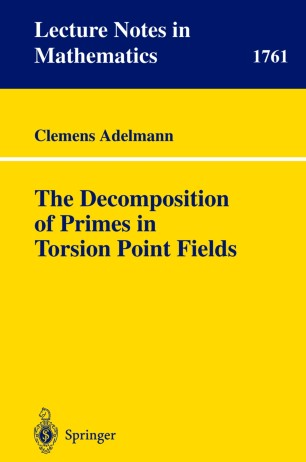# The Decomposition of Primes in Torsion Point FieldsBook

Part of the Lecture Notes in Mathematics book series (LNM, volume 1761)

1. Front Matter
Pages I-V
2. Pages 1-4
3. Pages 5-24
4. Pages 25-39
5. Pages 41-58
6. Pages 59-86
7. Pages 87-106
8. Back Matter
Pages 107-140

### Introduction

It is an historical goal of algebraic number theory to relate all algebraic extensionsofanumber?eldinauniquewaytostructuresthatareexclusively described in terms of the base ?eld. Suitable structures are the prime ideals of the ring of integers of the considered number ?eld. By examining the behaviouroftheprimeidealswhenembeddedintheextension?eld,su?cient information should be collected to distinguish the given extension from all other possible extension ?elds. The ring of integers O of an algebraic number ?eld k is a Dedekind ring. k Any non-zero ideal in O possesses therefore a decomposition into a product k of prime ideals in O which is unique up to permutations of the factors. This k decomposition generalizes the prime factor decomposition of numbers in Z Z. In order to keep the uniqueness of the factors, view has to be changed from elements of O to ideals of O . k k Given an extension K/k of algebraic number ?elds and a prime ideal p of O , the decomposition law of K/k describes the product decomposition of k the ideal generated by p in O and names its characteristic quantities, i. e. K the number of di?erent prime ideal factors, their respective inertial degrees, and their respective rami?cation indices. Whenlookingatdecompositionlaws,weshouldinitiallyrestrictourselves to Galois extensions. This special case already o?ers quite a few di?culties.

### Keywords

algebra algebraic number theory elliptic curve invariant theory modular form number theory prime number torsion